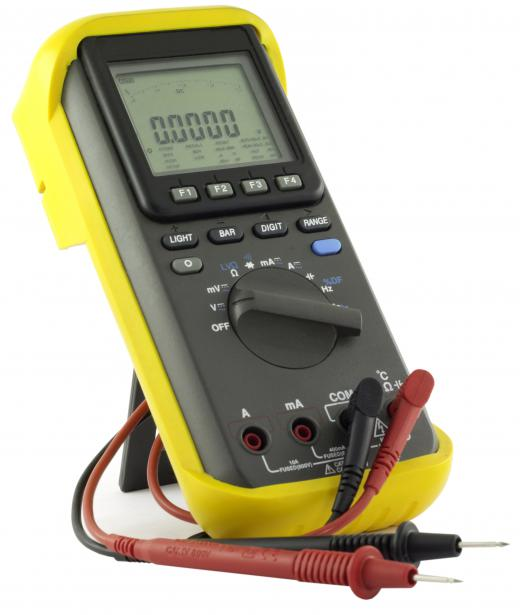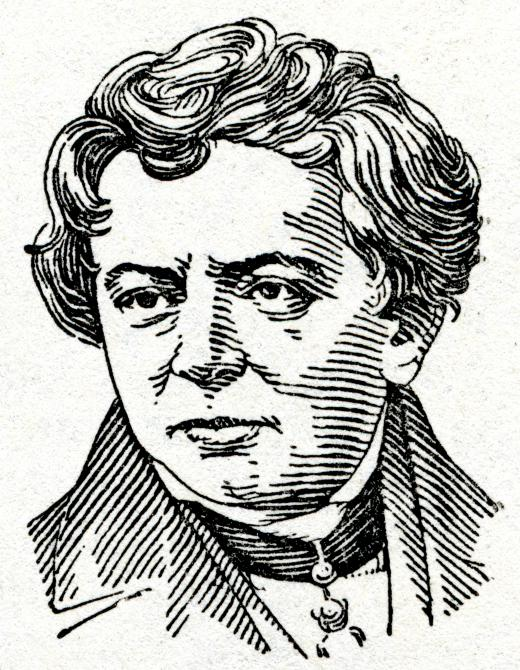# What is a Parallel Circuit?

B. Nolta
B. NoltaA multimeter, which can be used to test a parallel circuit.

A parallel circuit is one of the two basic types of electric circuit that can be found in electrical devices. "Circuit" refers to the total path of an electric current, or flow of electrical energy, and includes devices such as resistors, which control the flow of voltage, or difference in electrical charge, and capacitors, which store electrical charge. Circuits fall into one of two categories: series or parallel. In a series circuit, all the components of the circuit are lined up in a single path so that the current flows through each component in order.

In a parallel circuit, however, there are multiple pathways between the circuit’s beginning and end. As a result, since the current has more than one route to take, the circuit can still function if one path fails. This makes parallel circuits much more fail-resistant than series circuits which is why parallel circuits are common in everyday applications, such as household wiring. Regardless of how many different paths the circuit has, the total voltage stays the same, and all components of the circuit share the same common points. This set of common points is known as electrically common points. Every parallel circuit has two sets of them.Ohm's Law is named for 19th century German physicist Georg Ohm.

One thing to consider about parallel circuits is the current load that they carry. When a circuit has multiple paths for current, the circuit's total effective resistance drops. Since the voltage is equal to the current multiplied by the resistance — known as Ohm’s law, named for German physicist Georg Ohm — and the voltage does not change, this means the current has to increase. Thus, the more paths that a circuit has, the greater the current flow across each path will effectively become. This can lead to damage to the circuit or external equipment, which is why excessive use of outlet extenders or multi-plug inserts is considered hazardous. Parallel circuits are found in virtually all complex electrical devices. Many devices use both series and parallel circuits in conjoined and stand-alone configurations.

Another aspect of parallel circuits to keep in mind is that such circuits must be measured differently than series circuits. For example, when testing a parallel circuit using a voltmeter or multimeter, which tests multiple measurements, the multimeter must be connected in parallel to properly measure the voltage. Multiple branches means the load is distributed over more than one path, and measuring only one path will not present the full picture. If this isn’t done correctly, the measurement will be faulty, and the circuit may incorrectly be judged defective.

## You might also Likeanon973138

I've always wanted to know exactly what a parallel circuit is! This was just the information I needed to have!

KittenTucker

I've always wondered how my electronics were able to last so long without burning out; now I know. After reading this though, I found myself wondering what kinds of everyday things series circuits were used for, so I did a quick internet search, and found out that they're actually used in a ton of different things -- everything from Christmas tree light strings to batteries.

Isn't it crazy how such a simple technology can be used in so many different things?

JillT

Wow, this is really interesting. I've always wondered about the difference between these two circuits, so after reading this article I decided to do a little further research. I found a page that showed a picture of both a series circuit and a parallel circuit. I guess I'm just a super-visual person, but seeing the serial and parallel circuit pictures made the whole thing that much more understandable to me -- great job of explaining it in the article though!